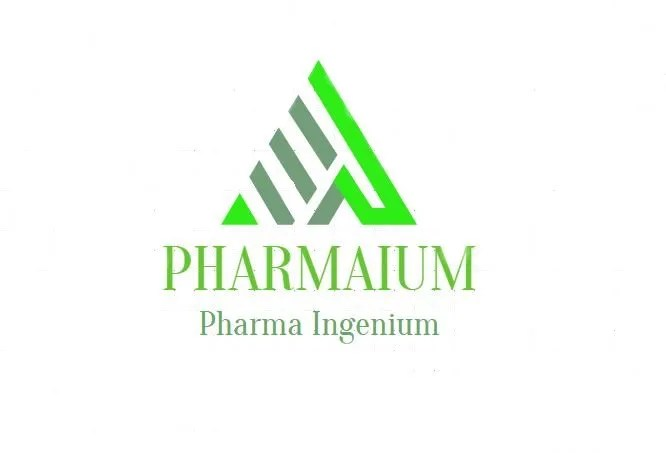# Quantification of the concentration of Cannabinoids in HPLCTRADELABOR has more than 20 years of experience in the control and treatment of air, working with an experienced and qualified technical staff and with the most advanced technology in this area, which together guarantee the quality of the services provided.

The cannabinoids produced by the plant are found in their acid form. When heat or UV lighting is applied these cannabinoides lose one molecule of CO2 and change into their neutral form. This process is called decarboxylation. Thus, when a cannabis sample is smoked or vaporised, a great portion of cannabinoids will be decarboxilated by the effect of the heat and will mutate into their neutral form. However, if the same sample is ingested, as no heat has been applied, the acidic cannabinoids will keep their original state.

The high performance liquid chromatography (HPLC) does not employ heat at any phase of the analysis and as a result, the samples are not decarboxylated. Therefore, when working with HPLC, if the cannabinoid content that any vegetable matter or extract has needs to be quantified, three different concentration results can be offered:

• % of Δ9-THC, CBD and CBG
• % of THCA, CBDA and CBGA
• % of THC Total, CBD Total and CBG Total

El THC Total, CBD Total and CBG Total are the maximum amounts that can be obtained from these cannabinoids if the sample is properly (or completely) decarboxilated.

THC Total percentage is the sum of the Δ9-THC percentage, plus the THCA percentage, multiplied by a conversion factor. Due to the fact that this factor is a number lower than 1, it is not surprising that the percentage of THC Total is less than the sum of Δ9-THC plus THCA, regardless of the conversion factor.

Knowing that the decarboxylation reaction is the transformation of THCA into THC through the loss of a carbon dioxide molecule (CO2), the conversion factor can be obtained in the following way:

When the THCA looses the CO2 molecule, it changes into THC, which is a smaller molecule, therefore, a less heavy molecule than THCA (THCA weighs 358 grams per mol, while THC weighs 314 grams per mol). Considering that loss, we will have 358/314=0.877 molecules of THC per molecule of THCA, thus, the correct way of calculating the percentage of THC Total in HPLC is:

% of THC Total = % of THC + (% of THCA x 0,877)

The same principle is applied for the calculation of the percentage of CBD Total and CBG Total. The formula for these cases is:

% of CBD Total = % of CBD + (% of CBDA x 0,877)
% of CBG Total = % of CBG + (% of CBGA x 0,878)

When samples are analysed by means of gas chromatography (GC), it is not necessary to apply this equation, because during the analysis they are exposed to very high temperatures. Thus, it is assumed that the decarboxilation reaction occurs entirely in the injector.
In the official method of “Regulation (EC) Nº 1177/2000 of 31st of May 2000” the injector is at 300ºC and the amount injected is very small. Therefore, it is understood that the decarboxilation reaction takes place in full.

# We build Industrial Site for Cannabis Oil Extraction. See more at:  PHARMAIUMThe fact that the decarboxilation of any kind of cannabis is not usually perfect must be taken into account. This could happen because, either it has not been possible to convert all the acids to a neutral state, because other products have been formed from deterioration, or even due to the partial evaporation of the cannabinoides. Therefore, the amount of neutral cannabinoids that will be obtained if the decarboxilation is performed could be slightly lower than the THC Total, CBD Total or CBG Total values obtained, given that these values express the maximum amount obtained in the case of 100% decarboxilation.

In the light of the above, we can conclude that the HPLC analysis, without conducting a previous decarboxilation of the samples, is the most precise technique in order to know the cannabinoids content in cannabis, as the result values will be the real values of the sample.

Continue at:  https://www.fundacion-canna.es/en/quantification-concentration-thc